# Electronics and Communication Engineering - Automatic Control Systems

### Exercise :: Automatic Control Systems - Section 2

36.

For an RLC series circuit Z(s) is of the form

 A.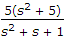B.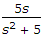C.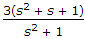D.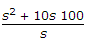Explanation: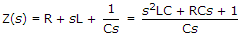.

37.

If feedback factor is β the overall gain of a closed loop system is approximately equal to

 A.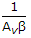B.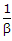C. β D. β

Explanation:

Closed loop gain =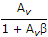. Since 1 << Av β, the gain is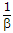.

38.

The functions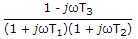and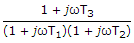have

 A. identical magnitude and phase curves B. identical magnitude curves but different phase curves C. different magnitude curves but identical phase curves D. different magnitude and phase curves

Explanation:

Magnitude are the same. i.e.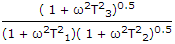.

However phase angles are different because the phase angle of 1 - jωT3 is negative but that of 1 - jωT3 is positive.

39.

For the op-amp circuit of the given figure, Eo(s)/Ei(s) =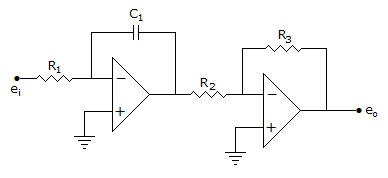A.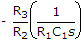B.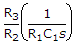C.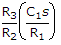D.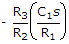Explanation: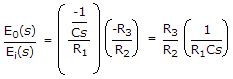40.

A unity feedback system has open loop transfer function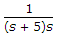The closed loop transfer function is

 A.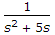B.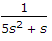C.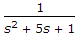D.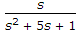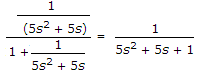.